Courses

# Test: The Triangle And Its Properties - 3

## 20 Questions MCQ Test Mathematics (Maths) Class 7 | Test: The Triangle And Its Properties - 3

Description
This mock test of Test: The Triangle And Its Properties - 3 for Class 7 helps you for every Class 7 entrance exam. This contains 20 Multiple Choice Questions for Class 7 Test: The Triangle And Its Properties - 3 (mcq) to study with solutions a complete question bank. The solved questions answers in this Test: The Triangle And Its Properties - 3 quiz give you a good mix of easy questions and tough questions. Class 7 students definitely take this Test: The Triangle And Its Properties - 3 exercise for a better result in the exam. You can find other Test: The Triangle And Its Properties - 3 extra questions, long questions & short questions for Class 7 on EduRev as well by searching above.
QUESTION: 1

### Find the measure of the angle x in the given figure.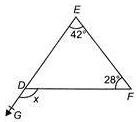Solution:

∠EFD+∠FED = x
(Exterior angle property of a triangle)
⇒ 28o+42= ∠x or ∠x = 70o

QUESTION: 2

### Which of the following options is INCORRECT?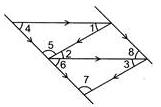Solution:

∠1 =∠2 and  ∠2 =∠3  [Alternate angles]
So, ∠1 =∠3  and  ∠1+∠4+∠5 = 180o  [Angle sum property]
Also, ∠8 = ∠6

QUESTION: 3

### In the figure (not drawn to scale), ADF and BEF are triangles and EC = ED, find y.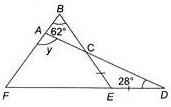Solution:

In ΔCED, CE=ED
∴ ∠EDC = ∠ECD
[Angles opposite to equal sides are equal] ⇒ ∠ECD = 28
Also,  ∠ECD = ∠BCA (Vertically opposite angles) ⇒ ∠BCA = 28
In ΔBCA, y = 62o+28o
Exterior angle property] ⇒ y = 90o

QUESTION: 4

In a ΔABC,which of the given condition holds?

Solution:
QUESTION: 5

In the figure (not drawn to scale), ABC is an equilateral triangle and ABD is an isosceles triangle with DA = DB, find x.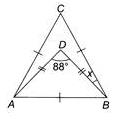Solution:

Since ABC is an equilateral triangle.
∴ ∠CAB =∠ABC =∠BCA = 60
And ∠DBA = ∠DAB = (60o−x)
[∵ DA = DB]
⇒ 2(60o−x)+88= 180o
⇒ 2(60o−x) = 92o
⇒ 60o−x = 46⇒ x = 14o

QUESTION: 6

ABC is an isosceles triangle with AB = AC and AD is altitude, then ____.

Solution:
QUESTION: 7

In the figure (not drawn to scale), ABCD is a square, ADE is an equilateral triangle and BFE is a straight line, find y.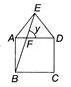Solution:

In ΔAEB,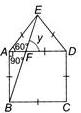And,  AE=AB ⇒ ∠ABE=∠AEB
[Angles opposite to equal sides are equal]
Now, ∠A+∠ABE+∠AEB=180  (Angle sum property)
⇒ 2∠AEB=180−150= 30o ⇒ ∠AEB = 15
Now, ∠E=60⇒ ∠DEF=60o−15= 45
∴ In ΔEFD, ∠DEF+∠EDF+∠EFD
= 180⇒ 45o+60o+y = 180
⇒ y = 180o−(45o+60o) = 75o

QUESTION: 8

Find the measure of the angle x in the given figure.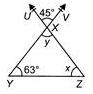Solution:

∠UXV = y (Vertically opposite angles)
∴ y = 45In ΔXYZ y+x+63o = 180
(Angle sum property)
⇒ 45o+x+63= 180o
⇒ x = 180o−(45o+63o
⇒  x= 180o−108= 72o

QUESTION: 9

The given figure shows three identical squares. Find x.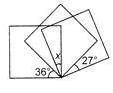Solution:

We have, ABCD, CEFG and CIHJ are all squares.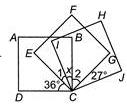So, ∠1+∠2+x=90???
(i) 36o+∠1+x=90o q ??.
(ii) x+∠2+27o=90??.
we get 36o+x+27o+(∠A+∠2+x)=180
⇒ 63o+x+90o=180
(From (i)) ⇒ x=180o−153= 27o

QUESTION: 10

In the figure (not drawn to scale), EFA is a right-angled triangle with ∠EFA=90o and FGB is an equilateral triangle, find  y−2x.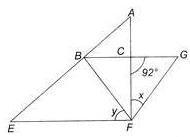Solution:

In ΔFGC, ∠CBF = 60o
(Angle of equilateral triangle)
∴  x+60o+92= 180o
⇒ x = 180o−152o=  28o
Now, In ΔBCF, ∠CBF = 60o
∠FCB = 180o−92o  (Linear pair)
⇒ ∠FCB=88o
∴ ∠BFC+88o+60o=180o
(Angle sum property)
⇒ ∠BFC=180o−148= 32o
And ∠AFE = 90o ⇒ y+32= 90o
⇒ y = 90o−32= 58o
∴ y−2x = 58o−2×28= 58o−56= 2o

QUESTION: 11

In the figure (not drawn to scale), ABC and DEF are two triangles, CA is parallel to FD and CFBE is a straight line. Find the value of x+y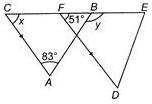Solution:

∠FCA = ∠BFD
(Corresponding angles)
⇒ x = 51o
Now, in ΔABC y = 51o+83o (Exterior angle property)
⇒ y = 134So, x+y = 51o+134= 185o

QUESTION: 12

In a ΔABC,if AB+BC = 10 cm, BC+CA = 12 cm,CA+AB = 16 cm, then the perimeter of the triangle is ____.

Solution:

It is given that,
AB+BC = 10cm   ?..(i)
BC+CA = 12cm    ?..(ii)
CA+AB = 16cm      ?..(iii)
Adding (i), (ii) and (iii); we get
2(AB+BC+CA) = 10+12+16
⇒ AB+BC+CA = 19cm

QUESTION: 13

In the figure, not drawn to scale, ACDF is a rectangle and BDE is a triangle. Find ∠BED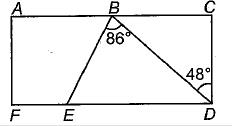Solution:

∠CDB+∠BDE = 90
(Angle of a rectangle)
⇒ 48o+∠BDE = 90o
⇒ ∠BDE = 90o−48= 42o
In ΔBED ∠EBD+∠BDE+∠BED = 180o
(Angle sum property)
⇒ 86o+42o+∠BED = 180
⇒ ∠BED = 180o−(86o+42o) = 52o

QUESTION: 14

In the figure, ABCD is a square, MDC is an equilateral triangle. Find the value of x.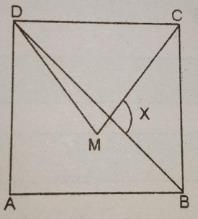Solution:

∆MDC is an equilateral triangle. therefore all the angles will be 60°.

angle MCB=BCD-MCD

=90-60 = 30°

NOW,

In ∆BCD,

BC=CD

Therefore angle XBC = 45°.

when angle sum is applied in ∆CBX, X=105°

QUESTION: 15

Find the measure of ∠LNM in the given figure.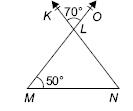Solution:

∠KLO = ∠MLN
∴ ∠MLN = 70o in ∠LMN,∠MLN+∠LNM+∠LMN = 180
(Angle sum property)
⇒ 70o+∠LNM+50o=180o

⇒ ∠LNM = 180o−(70o+50o) = 60o

QUESTION: 16

A 26 m long ladder reached a window 24 m from the ground on placing it against a wall. Find the distance of the foot of the ladder from the wall.

Solution:

In ΔPRQ,  PR= PQ2+QR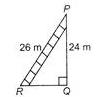(By Pythagoras theorem)
(26)=(24)2+QRor QR
= 676−576 = 100
⇒ QR = √100 ⇒ QR = 10
∴ The distance of the foot of the ladder from the wall is 10 m.

QUESTION: 17

A tree is broken at a height of 5 m from the ground and its top touches the ground at a distance of 12 m from the base of the tree. Find the original height of the tree.

Solution:

Let KB is original height of the tree. In ΔABC,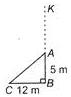AC= AB2+BC= 52+122 =25+144=169
∴ AC = √169 = 13m
KB = KA+AB = (13+5)m = 18m
∴ Original height of the tree is 18m.

QUESTION: 18

Aryan wants to plant a flower on the ground in the form of a rhombus. The diagonals of the rhombus measures 42 cm and 56 cm. Find the perimeter of the field.

Solution:

Since diagonals of a rhombus bisect each other at 90
Given: BD = 42 cm and AC = 56 cm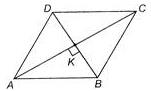∴ BK = 1/2 BD = 42/2 = 21cm
AK=1/2 AC = 56/2 = 28 cm
In ΔKAB,AB= AK2+BK= (28)2+(21)2=784+441=1225
∴ AB=√1225 = 35cm
∴  Perimeter of the field ABCD = 4×35 = 140 cm;

QUESTION: 19

A 34 m long ladder reached a window 16 m from the ground on placing it against a wall. Find the distance of the foot of the ladder from the wall.

Solution:

Let AB = length of ladder, AC = height of window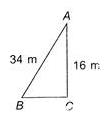In ΔABC, (AB)= (AC)2+(BC)2
⇒ (34)2  =(16)2+BC
or
BC= (34)2−(16)⇒ BC= 1156−256 = 900
∴ BC = √900 = 30m

QUESTION: 20

Mrs Kaushik gives a problem to her students. Find the perimeter of a rectangle whose length is 28 cm and diagonal is 35 cm. What will be the correct answer?

Solution:

ABCD is a rectangle,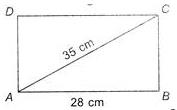In ΔACB, AC= AB2+BC2  (By Pythagoras theorem)
(35)= (28)2+BC2 or  BC= (35)2−(28)2
⇒ BC= 1225−784 ⇒ BC= 441
∴ BC=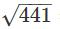=21cm
∴  Perimeter of rectangle
= 2×(28+21)cm = 2×(49)cm = 98cm